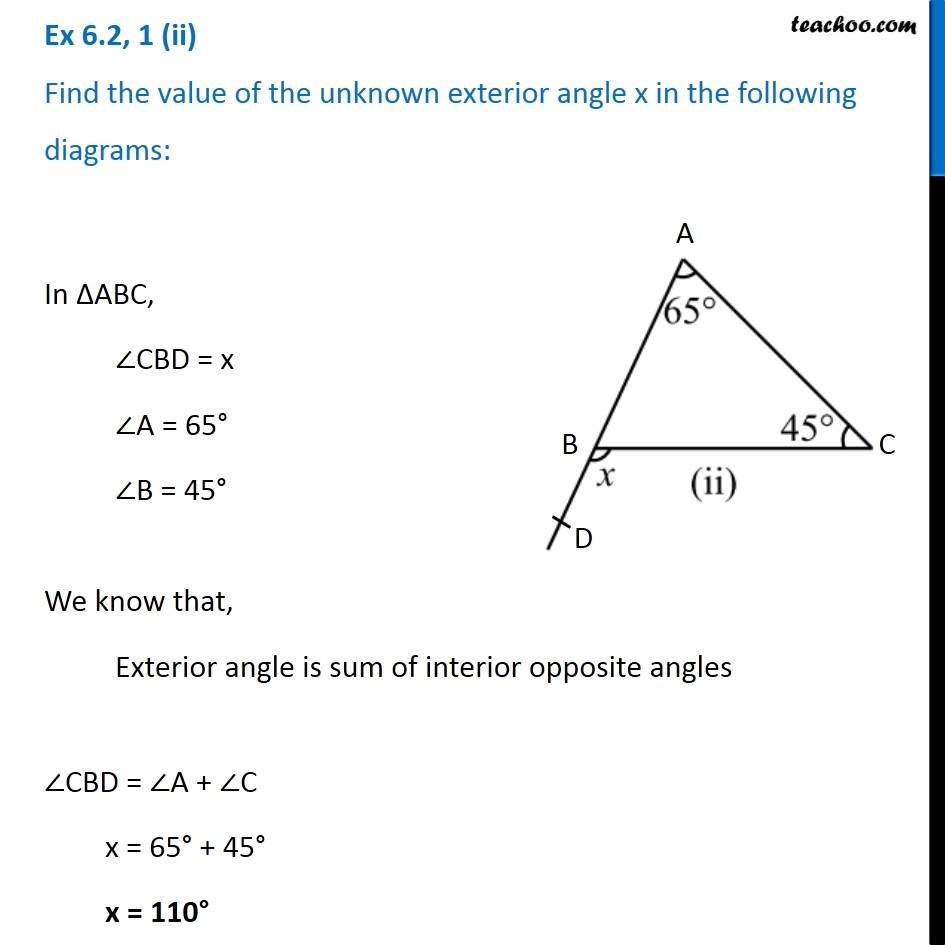1. Chapter 6 Class 7 Triangle and its Properties
2. Serial order wise
3. Ex 6.2

Transcript

Ex 6.2, 1 (ii) Find the value of the unknown exterior angle x in the following diagrams:In ∆ABC, ∠CBD = x ∠A = 65° ∠B = 45° We know that, Exterior angle is sum of interior opposite angles ∠CBD = ∠A + ∠C x = 65° + 45° x = 110°